# Expression Worksheets

#### What are Math Expressions?

An expression is a statement or sentence which consists of two or more numbers with at least one operator in the middle. The math operation can be in the form of addition, subtraction, multiplication, or division. For instance, a math expression can be in the form of 45 + 67 = 112. They can consist of several different types of terms and operations which can consist of numbers, operators, and or mathematical symbols. They are grouped together to show the value of some kind. We often use expressions to quickly translate math statements. Students can often confuse them with algebraic equations, but that is easy to spot because equations are two separate algebraic expressions that are linked by a equals symbol. You can characteristically identify equations by spotting the equals sign.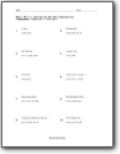###### Evaluating Variable Expressions

You are given an expression and the value of the variables involved. Just plug the value of those variables in the expression. Example: 6(2xy + 5yz + 7zx) ; x = -2, y = 3 , z = 1.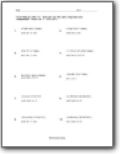###### Variable Expressions with Exponents

Evaluate expressions with some compounded exponents components. Example: a3 + 8b3 + 64c3 - 24abc; a = 3, b = -4, c = 2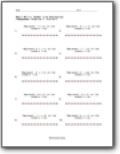###### Expressions on a Numbers Line

Demonstrate the value on numbers line with a little diagram. Example: Represent -7 - (-2) on the numbers line.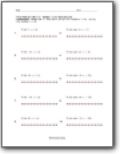###### Intermediate Skills

Evaluate using the numbers line. using the numbers line.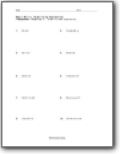###### Simplifying Expressions

Simplify each expression that is presented to you.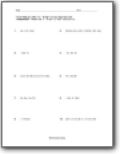###### Reduce the Expressions

Compound everything that you are given.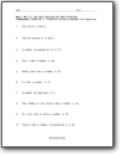###### Variable Expressions Word Problems

Translate the word problems into equations. Example: Four added to the three times a number is 40.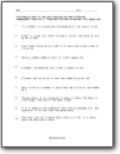###### Slightly Complex Variable Expressions Word Problems

We will step you the vocabulary and the length of the word problems. Example: If 3 is added to the numerator the fraction becomes 2/3. The numerator is 16 less than the denominator.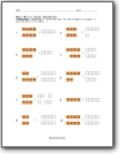###### Visual Expressions

Find the sum. Let one orange tile equal +1 and one white tile equal -1.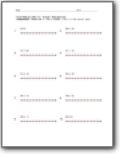###### Intermediate Skills

Use a number line to find sum of each. The sums can include negative numbers or operators.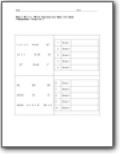###### Which Expressions Mean the Same

Group all these terms together as you see the similarities within the terms.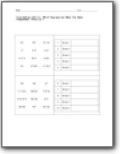###### Grouping Terms

Evaluate the terms and understand how they relate to one another.